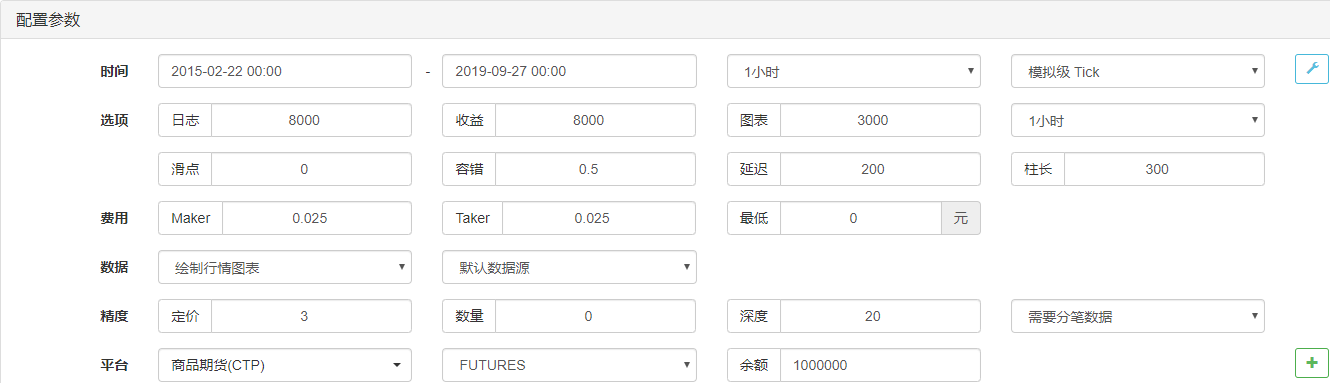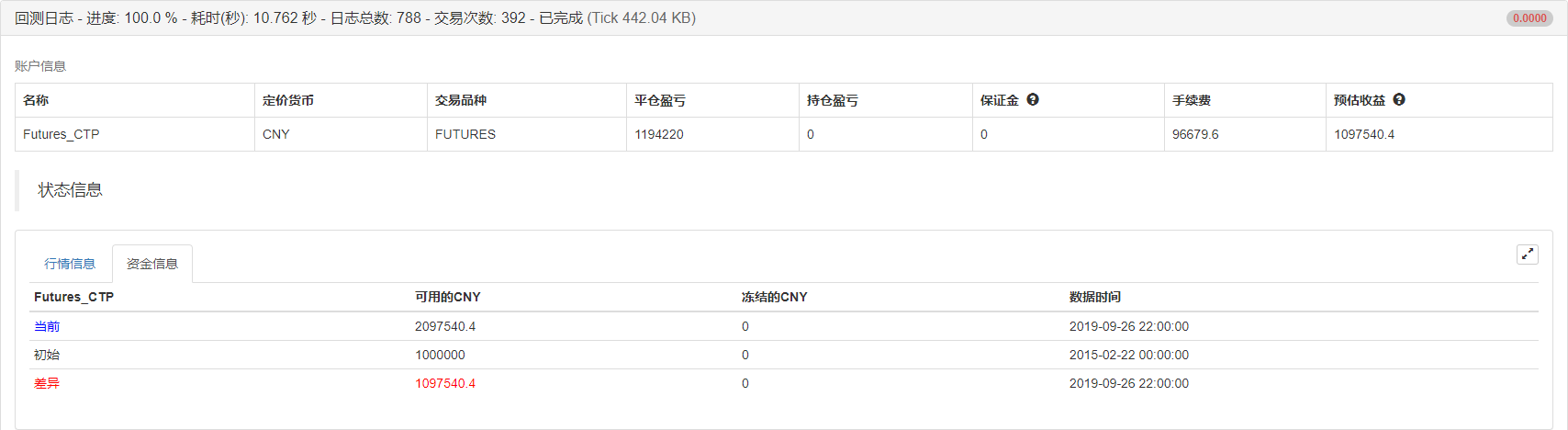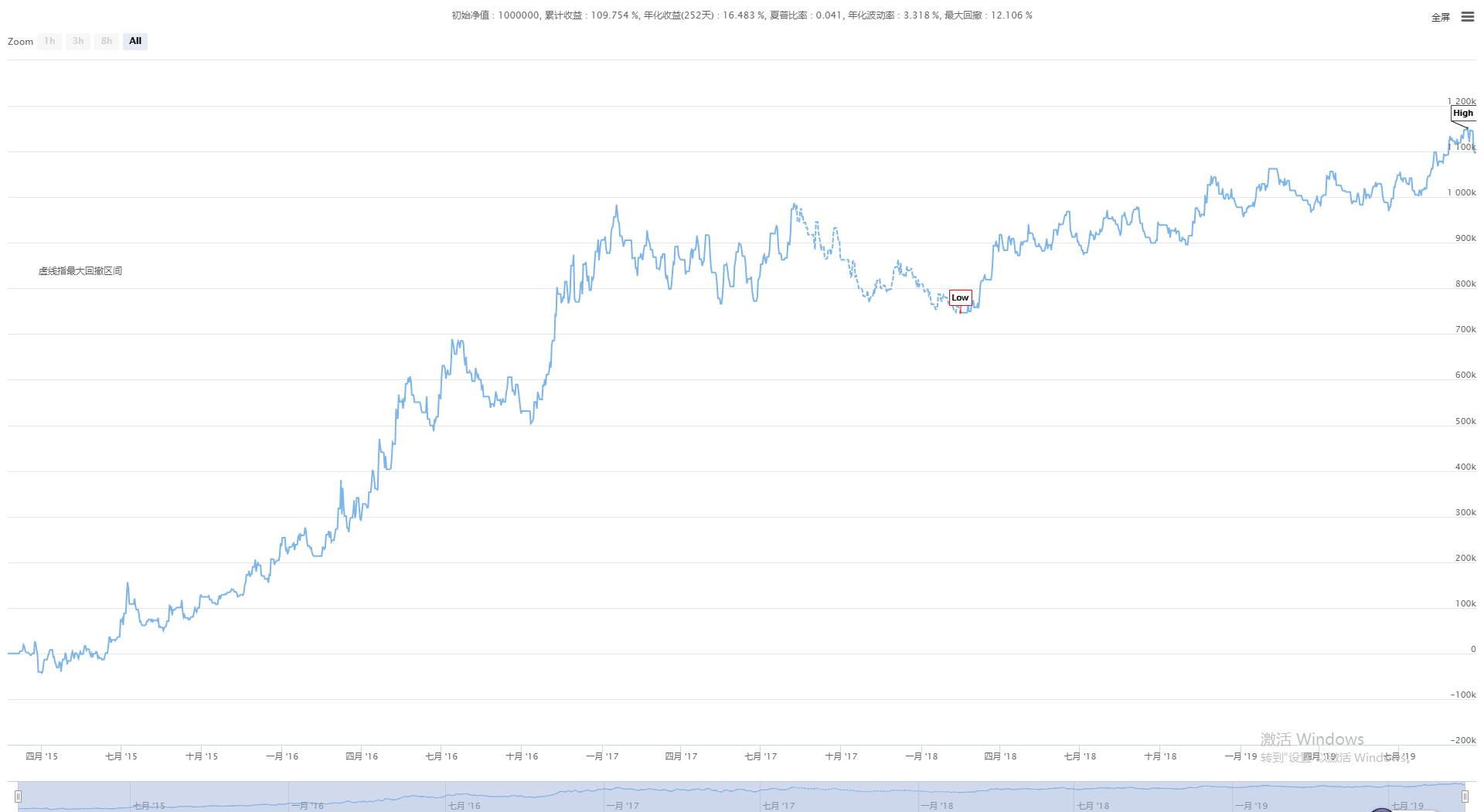# 抛物线转向SAR与价格高低点策略

Author: Hukybo, Created: 2019-09-28 16:11:09, Updated: 2019-12-16 10:42:24

#### 抛物线转向计算

• 如果SAR(t)大于t时间段的最低价L(t)，则发生跳转，在下一个时间段时进入跌势；

• 如果SAR(t)不大于t时间段的最低价L(t)，在下一个时间段时进入涨势；

• 极值Ep(t)等于最近N个时间段的最高价格；

``````SAR(t+1)=SAR(t)+Af(t)*(Ep(t) – SAR(t))
``````
• 如果SAR(t+1)大于t+1时间段的最低价L(t+1)，则发生跳转，在下一个时间段时进入跌势；

• 如果SAR(t+1)不大于t+1时间段的最低价L(t+1)，进入涨势下一步；并且，极值Ep(t+1)等于最近N个时间段的最高价格；

• 如果该时间段的最高价，即H(t+1)比前面N个时间段的最高价高，则AF(t+1)=AF(t)+0.02，否则，AF(t+1)=AF(t)。

• 如果SAR(t)小于t时间段的最高价H(t)，则发生跳转，在下一个时间段时进入涨势；

• 如果SAR(t)不小于t时间段的最高价H(t)，在下一个时间段时进入跌势；

• 极值Ep(t)等于最近N个时间段的最低价格；

SAR(t+1)=SAR(t)+Af(t)*(Ep(t) – SAR(t))

• 如果SAR(t+1)小于t+1时间段的最高价H(t+1)，则发生跳转，在下一个时间段时进入涨势；

• 如果SAR(t+1)不小于t+1时间段的最高价L(t+1)，进入跌势下一步；并且，极值Ep(t+1)等于最近N个时间段的最低价格；

• 如果该时间段的 最低价 L(t+1)，比前面N个时间段的最低价低，则AF(t+1)=AF(t)+0.02，否则，AF(t+1)=AF(t)。

#### 策略逻辑

• 多头开仓：抛物线为上涨，并且最高价大于前期高点
• 空头开仓：抛物线为下跌，并且最低价小于前期低点
• 多头止盈：抛物线为下跌，并且浮盈达到指定数额
• 空头止盈：抛物线为上涨，并且浮盈达到指定数额
• 多头止损：亏损达到指定额度
• 空头止损：亏损达到指定额度

#### 编写策略

``````N:=30;         // 最高/低价参数
SLOSS:=1;      // 止盈止损系数
FUND:=100000;  // 初始资金
``````

``````LOTS:=MAX(1,INTPART(FUND/(O*UNIT*0.1)));  // 计算下单量
SARLINE:=SAR(4,2,20);                     // 计算抛物线转向指标
B1:=SARLINE>0;                            // 判断是否上涨趋势
S1:=SARLINE<0;                            // 判断是否下跌趋势
B2:=HIGH>=HHV(CLOSE,N);                   // 判断最高价是否大于前期高点
S2:=LOW<=LLV(CLOSE,N);                    //  判断最低价是否小于前期低点
``````

``````BARPOS>N AND B1 AND B2,BK(LOTS);                        // 开多单
BARPOS>N AND S1 AND S2,SK(LOTS);                        // 开空单
S1 AND S2 AND BKHIGH>BKPRICE*(1+0.01*SLOSS),SP(BKVOL);  // 多单止盈
B1 AND B2 AND SKLOW<SKPRICE*(1-0.01*SLOSS),BP(SKVOL);   // 空单止盈
C<BKPRICE*(1-SLOSS*0.01),SP(BKVOL);                     // 多单止损
C>SKPRICE*(1+SLOSS*0.01),BP(SKVOL);                     // 空单止损
``````

#### 策略回测

• 交易品种：螺纹钢指数
• 时间：2015年02月22日~2019年09月27日
• 周期：一小时
• 滑点：开平仓各2跳
• 手续费：交易所2倍绩效报告资金曲线#### 完整策略代码

``````(*backtest
start: 2015-02-22 00:00:00
end: 2019-09-27 00:00:00
period: 1h
exchanges: [{"eid":"Futures_CTP","currency":"FUTURES"}]
args: [["ContractType","rb000",126961]]
*)

N:=30;         // 最高/低价参数
SLOSS:=1;      // 止盈止损系数
FUND:=100000;  // 初始资金

LOTS:=MAX(1,INTPART(FUND/(O*UNIT*0.1)));  // 计算下单量
SARLINE:=SAR(4,2,20);                     // 计算抛物线转向指标
B1:=SARLINE>0;                            // 判断是否上涨趋势
S1:=SARLINE<0;                            // 判断是否下跌趋势
B2:=HIGH>=HHV(CLOSE,N);                   // 判断最高价是否大于前期高点
S2:=LOW<=LLV(CLOSE,N);                    //  判断最低价是否小于前期低点

BARPOS>N AND B1 AND B2,BK(LOTS);                        // 开多单
BARPOS>N AND S1 AND S2,SK(LOTS);                        // 开空单
S1 AND S2 AND BKHIGH>BKPRICE*(1+0.01*SLOSS),SP(BKVOL);  // 多单止盈
B1 AND B2 AND SKLOW<SKPRICE*(1-0.01*SLOSS),BP(SKVOL);   // 空单止盈
C<BKPRICE*(1-SLOSS*0.01),SP(BKVOL);                     // 多单止损
C>SKPRICE*(1+SLOSS*0.01),BP(SKVOL);                     // 空单止损
``````

#### 结尾

More

Hukybo 暂时还没有JavaScript或者Python的版本，不过你可以根据上面的My语言代码翻译，关键函数可以使用Ta-Lib库。

bwxiaok 求问大佬，有JS或者python版本吗？谢谢